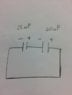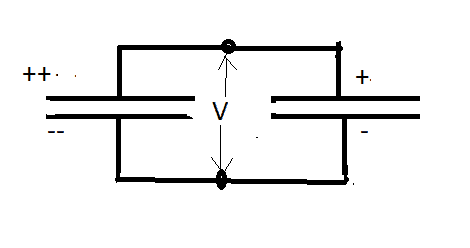# Capacitors in series

Romain Nzebele
Homework Statement:
A 25.0-mF capacitor and a 40.0-mF capacitor are charged by being connected across separate 50.0-V batteries. (a) Determine the resulting charge on each capacitor. (b) The capacitors are then disconnected from their batteries and connected to each other, with each negative plate connected to the other positive plate. What is the final charge of each capacitor?
Relevant Equations:
C=Q/V
I have no problems with part a). I used the formula for capacitance and determined the charges to be 0.00125 coulombs and 0.002 coulombs. The solution in the book is the same. For part b) my initial thought was that the charges will redistribute themselves so that each capacitor get the same charge. So I wrote that each capacitor will have (0.00125+0.002)/2=0.001625 coulombs. The solution in the book is very different.

Draw a diagram for part b. What can you say about the voltage of C1 and C2.

Romain Nzebele
Draw a diagram for part b. What can you say about the voltage of C1 and C2.
In my opinion the voltage across C1 remains 50V, across C2 remains 50V. By the way these capacitances are in microfarad (not in millifarad).

#### Attachments

•Image.jpeg
28.8 KB · Views: 102
Why do you say it is still 50V. If that is true then the charges on each respective capacitor would have to remain as they were before being connected.

Romain Nzebele
Why do you say it is still 50V. If that is true then the charges on each respective capacitor would have to remain as they were before being connected.
Then the Voltage across each resistor will change accordingly to have 0.001625 coulombs? I don’t see the point you are trying to make.

You connect two capacitors plus to minus. What do the charges do? What is the constraint on what they do?

Note that I am only suppose to lead you to the solution not tell you it.

Romain Nzebele
You connect two capacitors plus to minus. What do the charges do? What is the constraint on what they do?

Note that I am only suppose to lead you to the solution not tell you it.
I think charges will move until an equilibrium is achieved. The only way I see that equilibrium happening is if each capacitor gets an equal amount of charges. Is there another way to reach the equilibrium state?

Yes. The charges must redistribute according to the constraint that the voltage across each capacitor is the same.

Romain Nzebele
Yes. The charges must redistribute according to the constraint that the voltage across each capacitor is the same.
Is this something I have to commit to memory or can you tell me why the voltage must be the same? I thought in series, only charges and the current are the same.

Note that they can also be viewed as being in parallel, each capacitor shares a common node or point.

Romain Nzebele
Note that they can also be viewed as being in parallel, each capacitor shares a common node or point.
Can you please make a diagram of the circuit? I really do not see them in parallel.Romain Nzebele
Sorry but this confuses me more than it helps. I will stop here because I do not want this problem to make me reconsider my knowledge on parallel and series circuits.

Don't disengage. The circuit is both series and parallel. When viewed as parallel the two capacitors must have the same voltage. The charges on the shared connects must distribute in such a way as to fulfill this condition.

before the capacitors are connected they are charge to the same voltage. Because they have different capacitance the charges are different.The capacitors are now connected so the positive side of one is connected to the negative side of the other to form a completed circuit.The charges redistribute. (Note that the sum of the charges on the plates when connected must equal the sum of the charges on the separated plates before being connected) Even though there is nothing else connected to the capacitors at the dots you should recognize that they are in parallel which means they must have the same potential drop across them. Thus the charges on each capacitor must be different.

Note also that this pair of capacitor can be replace by a single one whose capacitance is the sum of the two capacitors with a charge on it equal to the sum of the charges on each capacitor.